### ISFORMULA Function Examples – Excel & Google Sheets

This tutorial demonstrates how to use the Excel ISFORMULA Function in Excel to test if a cell contains a formula.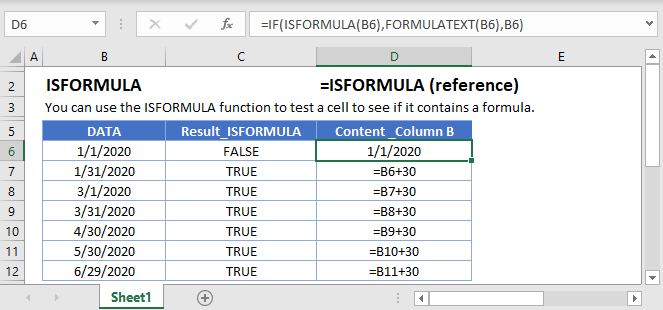## ISFORMULA Function Overview

The ISFORMULA Function Test if cell is a formula. Returns TRUE or FALSE.

To use the ISFORMULA Excel Worksheet Function, select a cell and type: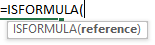(Notice how the formula inputs appear)

### ISFORMULA Function Syntax and Inputs:

`=ISFORMULA(reference)`

Cell Reference – The cell reference to the cell that you wish to test.

AutoMacro - VBA Code Generator

## How to use the ISFORMULA Function

The ISFORMULA Function tests if a cell contains a formula. If the cell contains a formula it returns TRUE, otherwise it returns FALSE.

`=ISFORMULA(A2)`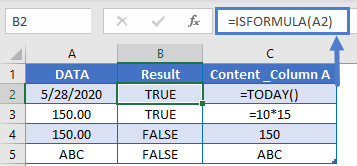### If Is Formula

Usually, after performing a logical test, you will want to do something based on the result of that test. The IF Function can be used to perform different actions if a statement is evaluated as TRUE or FALSE. Let’s use it with the ISFORMULA Function.

`=IF(ISFORMULA(A2),"formula", "not formula")`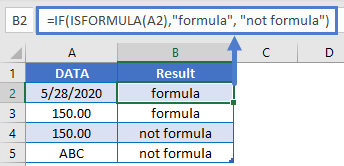### FORMULATEXT Function

The FORMULATEXT Function converts a cell formula to text. However, if the cell does not contain a formula, FORMULATEXT will throw an error. This is a great opportunity to use the ISFORMULA Function.

`=IF(ISFORMULA(A2),"formula", "not formula")`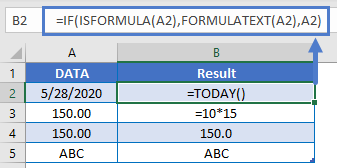In this example, we use the ISFORMULA Function to test if a cell contain a formula. If so, it will output the formula as text with the FORMULATEXT Function. If not, it will output the cell value.

### If Is Not Formula

The NOT Function inverts logical results. It changes TRUE to FALSE and FALSE to TRUE. Here we can use NOT, alongside the ISFORMULA and IF Functions.

`=IF(NOT(ISFORMULA(A2)),"not formula", "formula")`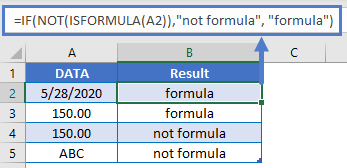### Other Logical Functions

Excel / Google Sheets contain many other logical functions to perform other logical tests. Here is a list:

IF / IS Functions
iferror
iserror
isna
iserr
isblank
isnumber
istext
isnontext
isformula
islogical
isref
iseven
isodd

## ISFORMULA in Google Sheets

The ISFORMULA Function works exactly the same in Google Sheets as in Excel: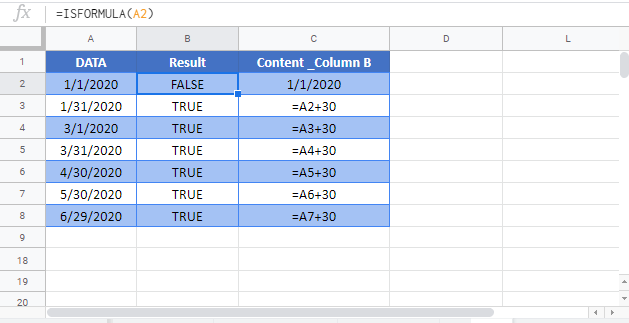## Excel Practice Worksheet

Practice Excel functions and formulas with our 100% free practice worksheets!

• Automatically Graded Exercises
• Learn Excel, Inside Excel!